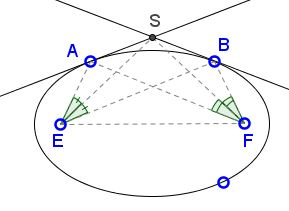# Angle Bisectors in Ellipse

Let A and B be two points on an ellipse with foci E and F. The tangents to the ellipse at A and B meet in S. Prove that ∠AES = ∠SEB. In words, the point of intersection of two tangents to an ellipse lies on the bisector of the angle formed by joining a focus to the points of tangency.

Proof### Conic Sections > EllipseLet A and B be two points on an ellipse with foci E and F. The tangents to the ellipse at A and B meet in S. Prove that ∠AES = ∠SEB. In words, the point of intersection of two tangents to an ellipse lies on the bisector of the angle formed by joining a focus to the points of tangency.### Proof

For a proof, reflect F in the two tangents to obtain points M and N. Because of the reflective property of ellipse, B is collinear with E, N and A with E, M.From the definition of ellipse, EA + FA = EB + FB. Since M and N are reflections of F, FB = BN and FA = AM, implying

 EM = EA + AM = EA + FA = EB + FB = EB + BN = EN.

It follows that ΔMEN is isosceles with EM = EN.

On the other hand, in ΔMFN, tangents AS and BS are the perpendicular bisectors of sides FN and FM. They meet in the circumcenter of ΔMFN, which also lies on the perpendicular bisector of the third side MN. By the construction, S is the point of intersection of the two tangents. Thus S is also the circumcenter of ΔMFN and lies on the perpendicular bisector of MN.

Now return to ΔMEN. Since it is isosceles with base MN, the perpendicular bisector of MN passes through E and plays the role of the bisector of ∠MEN which thus passes through S. But MEN and AEB is one and the same angle, and we are done.

(I thank Hubert Shutrick for his selfless help.)### Conic Sections > Ellipse### Angle Bisector

• Angle Bisector
• Angle Bisector Theorem
• Angle Bisectors in Ellipse II
• Angle Bisector in Equilateral Trapezoid
• Angle Bisector in Rectangle
• Property of Angle Bisectors
• Property of Angle Bisectors II
• A Property of Angle Bisectors III
• External Angle Bisectors
• Projections on Internal and External Angle Bisectors
• Angle Bisectors On Circumcircle
• Angle Bisectors in a Quadrilateral - Cyclic and Otherwise
• Problem: Angle Bisectors in a Quadrilateral
• Triangle From Angle Bisectors
• Property of Internal Angle Bisector - Hubert Shutrick's PWW
• Angle Bisectors Cross Circumcircle
• For Equality Choose Angle Bisector
•